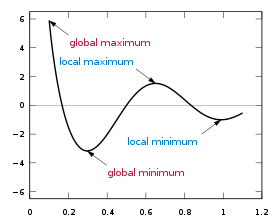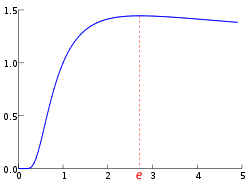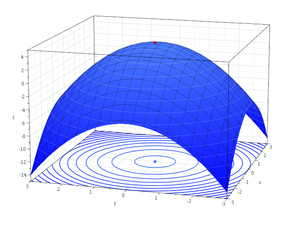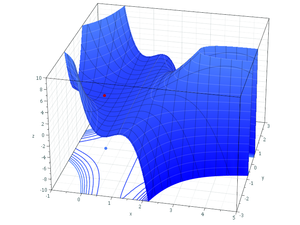# Maxima and minima

Maxima and minimaLocal and global maxima and minima for cos(3πx)/x, 0.1≤x≤1.1

In mathematics, the maximum and minimum (plural: maxima and minima) of a function, known collectively as extrema (singular: extremum), are the largest and smallest value that the function takes at a point either within a given neighborhood (local or relative extremum) or on the function domain in its entirety (global or absolute extremum).

More generally, the maximum and minimum of a set (as defined in set theory) are the greatest and least element in the set. Unbounded infinite sets such as the set of real numbers have no minimum and maximum.

To locate extreme values is the basic objective of optimization.

## Analytical definition

A real-valued function f defined on a real line is said to have a local (or relative) maximum point at the point x, if there exists some ε > 0 such that f(x) ≥ f(x) when |xx| < ε. The value of the function at this point is called maximum of the function. Similarly, a function has a local minimum point at x, if f(x) ≤ f(x) when |xx| < ε. The value of the function at this point is called minimum of the function.

A function has a global (or absolute) maximum point at x if f(x) ≥ f(x) for all x. Similarly, a function has a global (or absolute) minimum point at x if f(x) ≤ f(x) for all x. The global maximum and global minimum points are also known as the arg max and arg min: the argument (input) at which the maximum (respectively, minimum) occurs.

Restricted domains: There may be maxima and minima for a function whose domain does not include all real numbers. A real-valued function, whose domain is any set, can have a global maximum and minimum. There may also be local maxima and local minima points, but only at points of the domain set where the concept of neighborhood is defined. A neighborhood plays the role of the set of x such that |xx| < ε.

A continuous (real-valued) function on a compact set always takes maximum and minimum values on that set. An important example is a function whose domain is a closed (and bounded) interval of real numbers (see the graph above). The neighborhood requirement precludes a local maximum or minimum at an endpoint of an interval. However, an endpoint may still be a global maximum or minimum. Thus it is not always true, for finite domains, that a global maximum (minimum) must also be a local maximum (minimum).

## Finding functional maxima and minima

Finding global maxima and minima is the goal of mathematical optimization. If a function is continuous on a closed interval, then by the extreme value theorem global maxima and minima exist. Furthermore, a global maximum (or minimum) either must be a local maximum (or minimum) in the interior of the domain, or must lie on the boundary of the domain. So a method of finding a global maximum (or minimum) is to look at all the local maxima (or minima) in the interior, and also look at the maxima (or minima) of the points on the boundary; and take the biggest (or smallest) one.

Local extrema can be found by Fermat's theorem, which states that they must occur at critical points. One can distinguish whether a critical point is a local maximum or local minimum by using the first derivative test or second derivative test.

For any function that is defined piecewise, one finds a maxima (or minima) by finding the maximum (or minimum) of each piece separately; and then seeing which one is biggest (or smallest).

## ExamplesThe global maximum of$\sqrt[x]{x}$ occurs at x = e.
• The function x2 has a unique global minimum at x = 0.
• The function x3 has no global minima or maxima. Although the first derivative (3x2) is 0 at x = 0, this is an inflection point.
• The function$\sqrt[x]{x}$ has a unique global maximum at x = e. (See figure at right)
• The function x-x has a unique global maximum over the positive real numbers at x = 1/e.
• The function x3/3 − x has first derivative x2 − 1 and second derivative 2x. Setting the first derivative to 0 and solving for x gives stationary points at −1 and +1. From the sign of the second derivative we can see that −1 is a local maximum and +1 is a local minimum. Note that this function has no global maximum or minimum.
• The function |x| has a global minimum at x = 0 that cannot be found by taking derivatives, because the derivative does not exist at x = 0.
• The function cos(x) has infinitely many global maxima at 0, ±2π, ±4π, …, and infinitely many global minima at ±π, ±3π, ….
• The function 2 cos(x) − x has infinitely many local maxima and minima, but no global maximum or minimum.
• The function cos(3πx)/x with 0.1 ≤ x ≤ 1.1 has a global maximum at x = 0.1 (a boundary), a global minimum near x = 0.3, a local maximum near x = 0.6, and a local minimum near x = 1.0. (See figure at top of page.)
• The function x3 + 3x2 − 2x + 1 defined over the closed interval (segment) [−4,2] has two extrema: one local maximum at x = −1−√153, one local minimum at x = −1+√153, a global maximum at x = 2 and a global minimum at x = −4.

## Functions of more than one variable

For functions of more than one variable, similar conditions apply. For example, in the (enlargeable) figure at the right, the necessary conditions for a local maximum are similar to those of a function with only one variable. The first partial derivatives as to z (the variable to be maximized) are zero at the maximum (the glowing dot on top in the figure). The second partial derivatives are negative. These are only necessary, not sufficient, conditions for a local maximum because of the possibility of a saddle point. For use of these conditions to solve for a maximum, the function z must also be differentiable throughout. The second partial derivative test can help classify the point as a relative maximum or relative minimum.

In contrast, there are substantial differences between functions of one variable and functions of more than one variable in the identification of global extrema. For example, if a bounded differentiable function f defined on a closed interval in the real line has a single critical point, which is a local minimum, then it is also a global minimum (use the intermediate value theorem and Rolle's theorem to prove this by reductio ad absurdum). In two and more dimensions, this argument fails, as the function$f(x,y)= x^2+y^2(1-x)^3,\qquad x,y\in\mathbb{R},$

shows. Its only critical point is at (0,0), which is a local minimum with ƒ(0,0) = 0. However, it cannot be a global one, because ƒ(4,1) = −11.The global maximum is the point at the topCounterexample

## In relation to sets

Maxima and minima are more generally defined for sets. In general, if an ordered set S has a greatest element m, m is a maximal element. Furthermore, if S is a subset of an ordered set T and m is the greatest element of S with respect to order induced by T, m is a least upper bound of S in T. The similar result holds for least element, minimal element and greatest lower bound.

In the case of a general partial order, the least element (smaller than all other) should not be confused with a minimal element (nothing is smaller). Likewise, a greatest element of a partially ordered set (poset) is an upper bound of the set which is contained within the set, whereas a maximal element m of a poset A is an element of A such that if mb (for any b in A) then m = b. Any least element or greatest element of a poset is unique, but a poset can have several minimal or maximal elements. If a poset has more than one maximal element, then these elements will not be mutually comparable.

In a totally ordered set, or chain, all elements are mutually comparable, so such a set can have at most one minimal element and at most one maximal element. Then, due to mutual comparability, the minimal element will also be the least element and the maximal element will also be the greatest element. Thus in a totally ordered set we can simply use the terms minimum and maximum. If a chain is finite then it will always have a maximum and a minimum. If a chain is infinite then it need not have a maximum or a minimum. For example, the set of natural numbers has no maximum, though it has a minimum. If an infinite chain S is bounded, then the closure Cl(S) of the set occasionally has a minimum and a maximum, in such case they are called the greatest lower bound and the least upper bound of the set S, respectively..

Wikimedia Foundation. 2010.

### Look at other dictionaries:

• Maxima — may refer to: Maxima and minima, the highest and lowest values (points) of a function in calculus Maxima (comics), a character in the DC comics universe Maxima, musical note value in mensural notation Maxima of Rome, early Christian saint and… …   Wikipedia

• Maxima Group — Type Private Industry Retail, general merchandise Founded Vilnius …   Wikipedia

• Minima and maxima (introduction) — This is an entry level discussion of an advanced topic. For more comprehensive and rigorous treatment, see minima and maxima or extreme value. The minimum of a set of numbers is the smallest value in that set. So, for the set of numbers… …   Wikipedia

• Mathematics and Physical Sciences — ▪ 2003 Introduction Mathematics       Mathematics in 2002 was marked by two discoveries in number theory. The first may have practical implications; the second satisfied a 150 year old curiosity.       Computer scientist Manindra Agrawal of the… …   Universalium

• Sample maximum and minimum — Box plots of the Michelson–Morley experiment, showing sample maximums and minimums. In statistics, the maximum and sample minimum, also called the largest observation, and smallest observation, are the values of the greatest and least elements of …   Wikipedia

• Numerical smoothing and differentiation — An experimental datum value can be conceptually described as the sum of a signal and some noise, but in practice the two contributions cannot be separated. The purpose of smoothing is to increase the Signal to noise ratio without greatly… …   Wikipedia

• Leibniz and Newton calculus controversy — The calculus controversy was an argument between seventeenth century mathematicians Isaac Newton and Gottfried Leibniz over who had first invented calculus. Newton claimed to have begun working on a form of the calculus (which he called the… …   Wikipedia

• Inclusive Growth, Full Employment and Structural Change — «Inclusive Growth» redirige aquí. Para otras acepciones, véase Inclusive Growth (desambiguación). Inclusive Growth, Full Employment and Structural Change Inclusive Growth, Full Employment and Struc …   Wikipedia Español

• Comb filter — In signal processing, a comb filter adds a delayed version of a signal to itself, causing constructive and destructive interference. The frequency response of a comb filter consists of a series of regularly spaced spikes, giving the appearance of …   Wikipedia

• Differential calculus — The graph of a function, drawn in black, and a tangent line to that function, drawn in red. The slope of the tangent line equals the derivative of the function at the marked point. Topics in Calculus …   Wikipedia